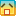## 【算法】八数码

• 内容
• 相关

``````1 2 3
x 4 6
7 5 8``````

``````1 2 3
4 5 6
7 8 x``````

``````1 2 3   1 2 3   1 2 3   1 2 3
x 4 6   4 x 6   4 5 6   4 5 6
7 5 8   7 5 8   7 x 8   7 8 x``````

#### 输入格式

``````1 2 3
x 4 6
7 5 8 ``````

#### 输入样例：

``2 3 4 1 5 x 7 6 8``

#### 输出样例

``19``

1 2 3
x 4 6
7 5 8 转化为 “123x46758”

#### 解题代码

``````/**
*
* 在一个 3×3 的网格中，1∼8 这 8 个数字和一个 x 恰好不重不漏地分布在这 3×3 的网格中。
*
* 例如：
*
* 1 2 3
* x 4 6
* 7 5 8
* 在游戏过程中，可以把 x 与其上、下、左、右四个方向之一的数字交换（如果存在）。
*
* 我们的目的是通过交换，使得网格变为如下排列（称为正确排列）：
*
* 1 2 3
* 4 5 6
* 7 8 x
* 例如，示例中图形就可以通过让 x 先后与右、下、右三个方向的数字交换成功得到正确排列。
*
* 交换过程如下：
*
* 1 2 3   1 2 3   1 2 3   1 2 3
* x 4 6   4 x 6   4 5 6   4 5 6
* 7 5 8   7 5 8   7 x 8   7 8 x
* 现在，给你一个初始网格，请你求出得到正确排列至少需要进行多少次交换。
*
* 输入格式
* 输入占一行，将 3×3 的初始网格描绘出来。
*
* 例如，如果初始网格如下所示：
*
* 1 2 3
* x 4 6
* 7 5 8
* 则输入为：1 2 3 x 4 6 7 5 8
*
* 输出格式
* 输出占一行，包含一个整数，表示最少交换次数。
*
* 如果不存在解决方案，则输出 −1。
*
* 输入样例：
* 2 3 4 1 5 x 7 6 8
* 输出样例
* 19
*
*/
public class Main {

public static int bfs(String start) {
Queue<String> queue =  new ArrayDeque();
HashMap<String , Integer> distances = new HashMap<>();
String end = "12345678x";
queue.offer(start);
distances.put(start,0);

int[] dx = {0, -1, 0, 1}, dy = {1, 0, -1, 0};
while(!queue.isEmpty()){
String t = queue.poll();
int distance = distances.get(t);
if(end.equals(t)){
return distance;
}
char[] cc = t.toCharArray();
int index = t.indexOf("x");
int x = index / 3, y = index % 3;
for(int i = 0; i < 4; i++){
int a = x + dx[i], b = y + dy[i];
if(a >= 0 && a < 3 && b >= 0 && b < 3){
//交换数字和x位置
char tt = cc[3 * a + b];
cc[index] = tt;
cc[3 * a + b] = 'x';
if(!distances.containsKey(String.valueOf(cc))){
distances.put(String.valueOf(cc),distance + 1);
}
//还原 - 留给下一个操作
char ttt = cc[index];
cc[index] = 'x';
cc[3 * a + b] = ttt;
}
}
}
return -1;
}

public static void main(String[] args) {
Scanner scanner = new Scanner(System.in);
String ss = scanner.nextLine();
String[] s = ss.split(" ");
String start = "";
for(int i = 0; i < s.length ; i++){
start += s[i];
}
System.out.println(bfs(start));
}
}``````

### 评论

1条评论
1.广西 广电宽带(全省通用)